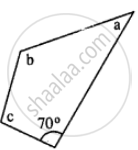# In the Given Figure : ∠B = 2a + 15 and ∠C = 3a + 5; Find the Values of B and C. - Mathematics

Sum

In the given figure : ∠b = 2a + 15 and ∠c = 3a + 5; find the values of b and c.#### Solution

∵ Sum of angles of quadrilateral = 360°

70° + a + 2a + 15 + 3a + 5 = 360°

6a + 90° = 360°

6a = 270°

a = 45°

∴ b = 2a + 15 = 2 x 45 + 15 = 105°

c = 3a + 5 = 3 x 45 + 5 = 140°

Hence ∠b and ∠c are 105° and 140°

Is there an error in this question or solution?
Chapter 16: Understanding Shapes - Exercise 16 (C) [Page 187]

#### APPEARS IN

Selina Concise Mathematics Class 8 ICSE
Chapter 16 Understanding Shapes
Exercise 16 (C) | Q 8 | Page 187
Selina Class 6 Mathematics